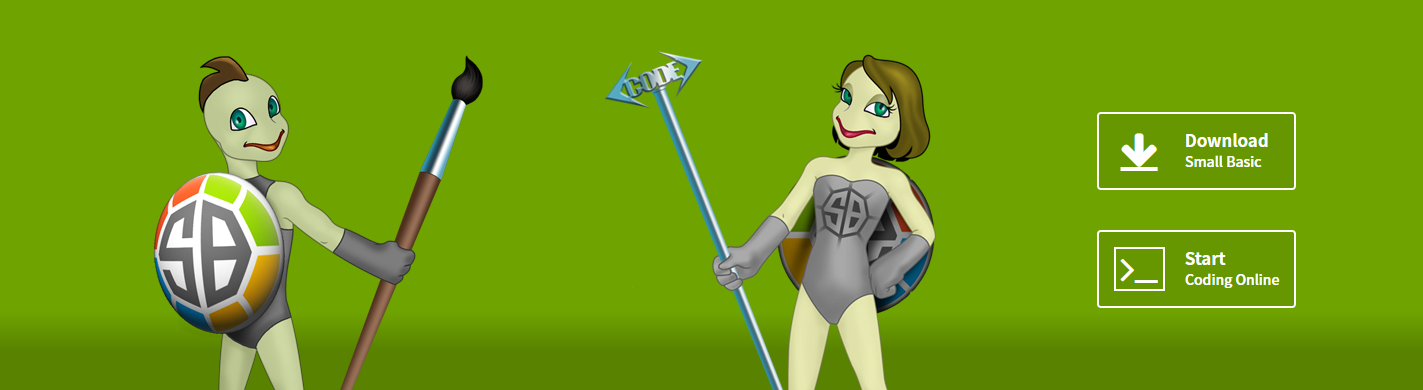## 為什麼是 Python，VBA 不香嗎？或 JS 不香嗎？

• 可以用 pandas 等著名的數據套件處理 Excel 資料。
• 可以在 Jupyter Notebook 環境或任何你喜愛的編輯器寫 Python 腳本，再也不用碰那陳年的 VBA 編輯器。
• 你的巨集腳本可以被版控、被集中整理，而不會隨著 Excel 檔案散落四方，因而享有所有版控的特性，多人協作、功能分支等等。
• 你還可以在 Python 腳本內透過 xlwings 調用 Excel 公式。

## 用 Python 操控 Excel

### 初始化與讀檔、存檔

``````import xlwings as xw

wb: xw.Book = app.books.open('example.xlsx')
sheet: xw.Sheet = wb.sheets

# handle the sheet

wb.save()
``````

### 選擇儲存格

``````# Excel 標示法－單格
sheet.range('A1')

# Excel 標示法－多格
sheet.range('A1:C3')

# 以 1 為首的 tuple (row, column) 標示法－單格
sheet.range((1,2)) # B1

# 以 1 為首的 tuple (row, column) 標示法－多格
sheet.range((1,2), (3,4)) # B1:D3
``````

### 儲存格的取值與賦值

``````>>> rng1 = sheet.range((1,2)) # B1
>>> rng1.value
'每次 Jira 的卡頓都令人抓狂'
>>> rng1.value = '用戰術上的勤奮掩蓋戰略上的怠惰'
>>> rng1.value
'用戰術上的勤奮掩蓋戰略上的怠惰'

>>> rng2 = sheet.range((1,2), (1,4)) # B1:D1
>>> rng2.value
['a', 'b', 'c']
>>> rng2.value = ['d', 'e', 'f']
>>> rng2.value
['d', 'e', 'f']

>>> rng3 = sheet.range((10,1), (11,3)) # A10:C11
>>> rng3.value
[['a', 'b', 'c'], [10.0, 20.0, 30.0]]
``````

### 小訣竅

• 一個 xlwings 的 `App` 可以開啟多份 Excel 檔案，可以互相剪剪貼貼。
• 也可以開多個 xlwings 的 `app`，此時他們會有各自的程序 ID。

## 結語

VB 大哥已死，小弟弟 Small Basic 又注定只能是玩具不能是工具，肩負著教育責任的 Small Basic 首頁卻有著奇葩的烏龜人：